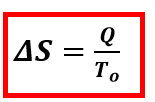Chemistry Practice Problems Entropy Practice Problems Solution: The element gallium (Ga) freezes at 29.8°C, and it...

🤓 Based on our data, we think this question is relevant for Professor Topich's class at VCU.

# Solution: The element gallium (Ga) freezes at 29.8°C, and its molar enthalpy of fusion is ΔH fus = 5.59kJ/mol.1. When molten gallium solidifies to Ga(s) at its normal melting point, is ΔS positive or negative?2. Calculate the value of ΔS when 70.0g of Ga(l) solidifies at 29.8°C. ΔS = ?

###### Problem

The element gallium (Ga) freezes at 29.8°C, and its molar enthalpy of fusion is ΔH fus = 5.59kJ/mol.

1. When molten gallium solidifies to Ga(s) at its normal melting point, is ΔS positive or negative?

2. Calculate the value of ΔS when 70.0g of Ga(l) solidifies at 29.8°C. ΔS = ?

###### Solution

At constant temperature, the change in entropy can be expressed as:Where: Q = heat , T = TemperatureView Complete Written Solution

Entropy

Entropy

#### Q. Predict which of the following compounds follow Trouton's rule:

Solved • Tue Jul 09 2019 20:45:21 GMT-0400 (EDT)

Entropy

#### Q. For each of the following pairs, predict which substance possesses the larger entropy per mole.Compare 1 mol of O2(g)  at 300 oC, 0.01 atm and 1 mol o...

Solved • Thu Jan 03 2019 09:45:17 GMT-0500 (EST)

Entropy

#### Q. In chemical kinetics, the entropy of activation is the entropy change for the process in which the reactants reach the activated complex.Predict whe...

Solved • Thu Jan 03 2019 09:45:17 GMT-0500 (EST)

Entropy

#### Q. For each of the following pairs, predict which substance has the higher entropy per mole at a given temperature.He(l) or He(g).

Solved • Thu Jan 03 2019 09:45:16 GMT-0500 (EST)